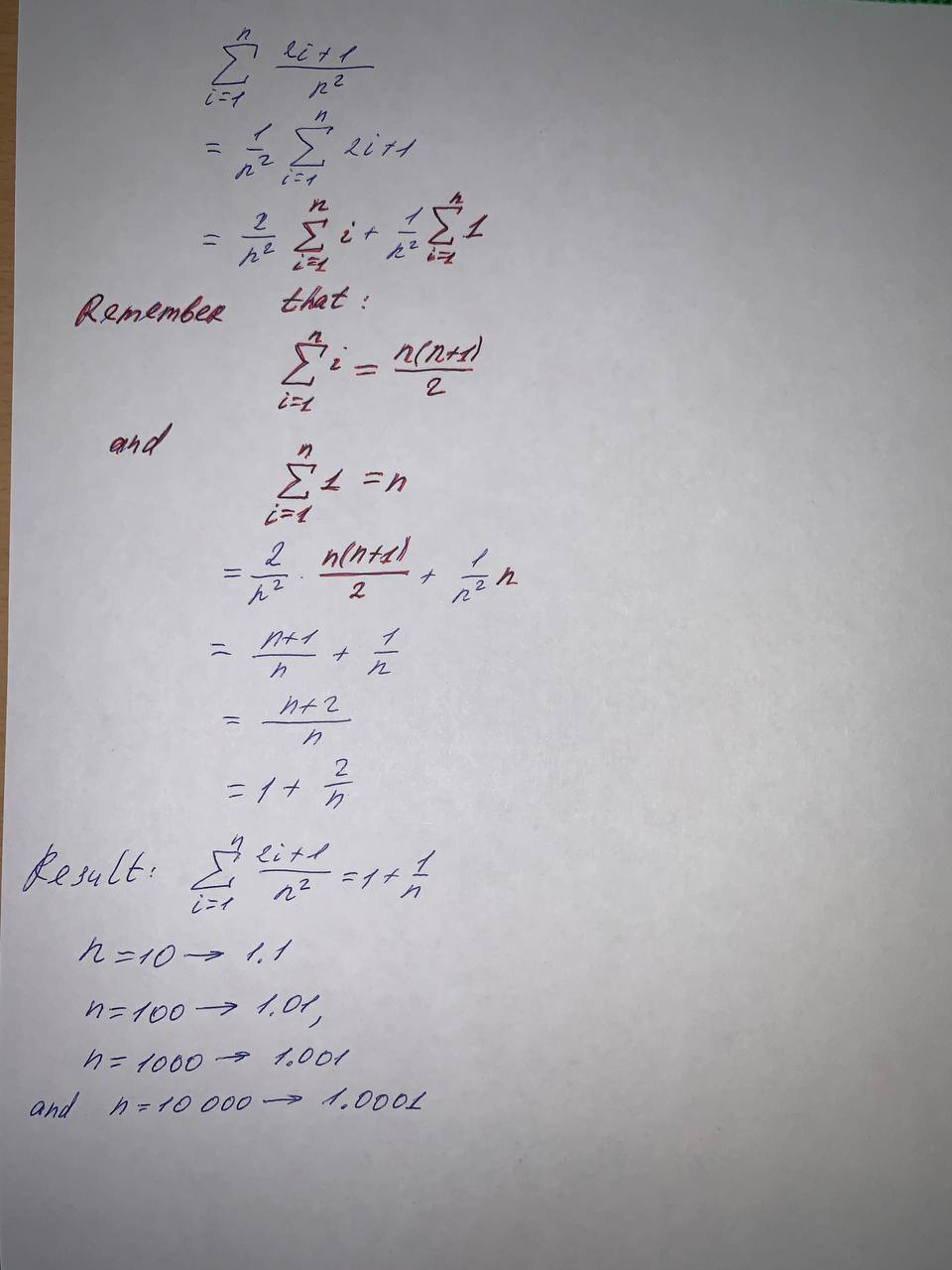2021-05-21

Use the summation formulas to rewrite the expression without the summation notation. Use the result to find the sums for n= 10, 100, 1000, and 10,000. $\sum _{i=1}^{n}\frac{2i+1}{{n}^{2}}$averes8..............................

Do you have a similar question?